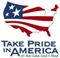Scientific Investigations Report 2008-5070

U.S. GEOLOGICAL SURVEY
Scientific Investigations Report 2008-5070

# Limitations of Water-Temperature Model

The accuracy of the water temperatures estimated by the SNTEMP model is limited by four types of error: model, data, parameter, and user errors.

Model error occurs when the equations used to represent physical processes are flawed or incomplete. This happens in all models in varying degrees because a model by definition is a simplified version of a real system. Model error can result from the use of an improper equation, use of the wrong numerical method to represent the equation, and calculation errors in the computer code that might make a model overly sensitive to certain input parameters. A potentially significant source of model error in SNTEMP is the method used to calculate daily maximum water temperature (Bartholow, 2000). The form of the equation used to calculate daily maximum water temperature (Theurer and others, 1984, equation II(116)) is the same as that used for daily mean water temperature (Theurer and others, 1984, equation II(115)). However, because of limitations in the model computer code, daily maximum air temperature cannot be used in place of the daily mean air temperature. Instead, a regression equation that includes relative humidity, wind speed, and solar radiation is used to predict the daily maximum air temperature, which is used as input into equation II(116). The coefficients for the regression equation (Theurer and others, 1984, table II.3 page II-32) are based on measurements collected throughout the country. A method exists to estimate regression coefficients by using local weather data (Bartholow, 2000). This was attempted by using data from the AgriMet weather station (Harrah). However, when the results from the regression equation were compared against measured data, the local coefficients did not predict daily maximum air temperature as well as the coefficients from table II.3 in the User’s Manual (Theurer and others, 1984).

Data error occurs when the input data used in the model do not accurately represent actual conditions. Input data for the Roza–Prosser Reach contained data errors because much of the input data were estimated.

Input flow data contain data errors for at least four reasons:

1. Flow estimates in all ungaged laterals (for example. streams, canals, drains, returns) were based on flow measurements from one USGS September 2005 seepage run. The proportion of flow in the laterals measured in September 2005 generally was assumed to be the same throughout the growing season but the actual proportion of flow varies over the growing season and adds error to water temperature estimates on any given day.
2. All flow estimates for the model were based on daily flow measurements made at 13 gages maintained by the Bureau of Reclamation. Data errors at these gages (especially during large runoff events) add data error to flow estimates.
3. Ground-water flow to or from a reach is based on estimated surface-water flows. When inflow to a reach is less than outflow, SNTEMP adds ground water to maintain the water balance in the reach. Therefore, errors in surface-water flow estimates are related to errors in ground-water estimates. The work required to characterize ground-water exchange more accurately in the Roza–Prosser Reach was beyond the scope of this project.

(4) Data error may occur because inflow to the Yakima River mainstem from holding ponds and oxbow lakes may not be accurately represented. The exchange between those bodies of water is not well understood. Therefore, greater data error could occur during low-flow conditions in the mainstem when inflow from ponds and oxbow lakes may be proportionally higher. Characterizing the flow exchange between the mainstem and adjacent ponds and lakes also was beyond the scope of this study.

Input water temperatures contain data errors because these data were generated using regression equations, which are approximations of actual conditions. Data also were not available to develop a regression equation for every inflowing lateral. Many laterals were assigned water temperature values from a nearby lateral (with a developed regression equation), which increases data error.

Input meteorology data contain data errors because actual conditions along the mainstem vary from conditions at the AgriMet weather station at Harrah. Differences between meteorological data at Harrah and at four USGS weather stations along the mainstem are discussed in the section, “Meteorology”.

Parameter error occurs when variables adjusted to calibrate a model are set to values that do not represent actual conditions. For example, Manning’s N can be adjusted to improve the agreement between measured and simulated water temperatures, but instead of obtaining a better representation of the system, the adjustment might be concealing poorly represented processes in the model that caused the poor agreement.

User error can occur when the user makes a mistake. This can happen when a user assumes a condition exists when in fact it does not or when a user makes a programming error or enters incorrect data.U.S. Department of the Interior | U.S. Geological Survey